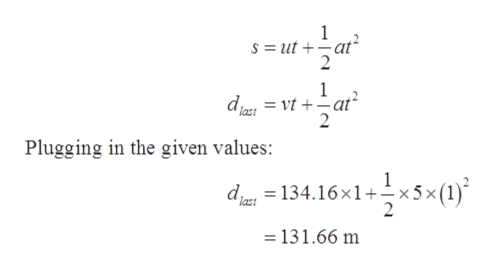# A plane accelerates from rest at a constant rate of 5.00 m/s2 along a runway that is 1800 m long. Assume that the plane reaches the required takeoff velocity at the end of the runway.What is the distance dlast traveled by the plane in the last second before taking off?

Question
30 views

A plane accelerates from rest at a constant rate of 5.00 m/s2 along a runway that is 1800 m long. Assume that the plane reaches the required takeoff velocity at the end of the runway.

What is the distance dlast traveled by the plane in the last second before taking off?

check_circle

star
star
star
star
star
1 Rating
Step 1

Given values:

Acceleration of the plan, a = 5.00 m /s2

Length of runway, S = 1800 m

Initial velocity of the plane, u = 0

Step 2

Let the required takeoff velocity be v

From third equation of motion:

Step 3

Distance travelled by the plane in the last second before taking off...help_outlineImage Transcriptionclose1 S = ut +at 1 = vtat last Plugging in the given values: 1 d134.16x1 x5x (1) =131.66 m fullscreen

### Want to see the full answer?

See Solution

#### Want to see this answer and more?

Solutions are written by subject experts who are available 24/7. Questions are typically answered within 1 hour.*

See Solution
*Response times may vary by subject and question.
Tagged in

### Physics